# 8.4 Rotation of axes  (Page 5/8)

 Page 5 / 8

Identify the conic for each of the following without rotating axes.

1. ${x}^{2}-9xy+3{y}^{2}-12=0$
2. $10{x}^{2}-9xy+4{y}^{2}-4=0$
1. hyperbola
2. ellipse

Access this online resource for additional instruction and practice with conic sections and rotation of axes.

## Key equations

 General Form equation of a conic section $A{x}^{2}+Bxy+C{y}^{2}+Dx+Ey+F=0$ Rotation of a conic section Angle of rotation

## Key concepts

• Four basic shapes can result from the intersection of a plane with a pair of right circular cones connected tail to tail. They include an ellipse, a circle, a hyperbola, and a parabola.
• A nondegenerate conic section has the general form $\text{\hspace{0.17em}}A{x}^{2}+Bxy+C{y}^{2}+Dx+Ey+F=0\text{\hspace{0.17em}}$ where $\text{\hspace{0.17em}}A,B\text{\hspace{0.17em}}$ and $\text{\hspace{0.17em}}C\text{\hspace{0.17em}}$ are not all zero. The values of $\text{\hspace{0.17em}}A,B,$ and $\text{\hspace{0.17em}}C\text{\hspace{0.17em}}$ determine the type of conic. See [link] .
• Equations of conic sections with an $\text{\hspace{0.17em}}xy\text{\hspace{0.17em}}$ term have been rotated about the origin. See [link] .
• The general form can be transformed into an equation in the $\text{\hspace{0.17em}}{x}^{\prime }\text{\hspace{0.17em}}$ and $\text{\hspace{0.17em}}{y}^{\prime }\text{\hspace{0.17em}}$ coordinate system without the $\text{\hspace{0.17em}}{x}^{\prime }{y}^{\prime }\text{\hspace{0.17em}}$ term. See [link] and [link] .
• An expression is described as invariant if it remains unchanged after rotating. Because the discriminant is invariant, observing it enables us to identify the conic section. See [link] .

## Verbal

What effect does the $\text{\hspace{0.17em}}xy\text{\hspace{0.17em}}$ term have on the graph of a conic section?

The $\text{\hspace{0.17em}}xy\text{\hspace{0.17em}}$ term causes a rotation of the graph to occur.

If the equation of a conic section is written in the form $\text{\hspace{0.17em}}A{x}^{2}+B{y}^{2}+Cx+Dy+E=0\text{\hspace{0.17em}}$ and $\text{\hspace{0.17em}}AB=0,$ what can we conclude?

If the equation of a conic section is written in the form $\text{\hspace{0.17em}}A{x}^{2}+Bxy+C{y}^{2}+Dx+Ey+F=0,$ and $\text{\hspace{0.17em}}{B}^{2}-4AC>0,$ what can we conclude?

The conic section is a hyperbola.

Given the equation $\text{\hspace{0.17em}}a{x}^{2}+4x+3{y}^{2}-12=0,$ what can we conclude if $\text{\hspace{0.17em}}a>0?$

For the equation $\text{\hspace{0.17em}}A{x}^{2}+Bxy+C{y}^{2}+Dx+Ey+F=0,$ the value of $\text{\hspace{0.17em}}\theta \text{\hspace{0.17em}}$ that satisfies $\text{\hspace{0.17em}}\mathrm{cot}\left(2\theta \right)=\frac{A-C}{B}\text{\hspace{0.17em}}$ gives us what information?

It gives the angle of rotation of the axes in order to eliminate the $\text{\hspace{0.17em}}xy\text{\hspace{0.17em}}$ term.

## Algebraic

For the following exercises, determine which conic section is represented based on the given equation.

$9{x}^{2}+4{y}^{2}+72x+36y-500=0$

${x}^{2}-10x+4y-10=0$

$AB=0,$ parabola

$2{x}^{2}-2{y}^{2}+4x-6y-2=0$

$4{x}^{2}-{y}^{2}+8x-1=0$

$AB=-4<0,$ hyperbola

$4{y}^{2}-5x+9y+1=0$

$2{x}^{2}+3{y}^{2}-8x-12y+2=0$

$AB=6>0,$ ellipse

$4{x}^{2}+9xy+4{y}^{2}-36y-125=0$

$3{x}^{2}+6xy+3{y}^{2}-36y-125=0$

${B}^{2}-4AC=0,$ parabola

$-3{x}^{2}+3\sqrt{3}xy-4{y}^{2}+9=0$

$2{x}^{2}+4\sqrt{3}xy+6{y}^{2}-6x-3=0$

${B}^{2}-4AC=0,$ parabola

$-{x}^{2}+4\sqrt{2}xy+2{y}^{2}-2y+1=0$

$8{x}^{2}+4\sqrt{2}xy+4{y}^{2}-10x+1=0$

${B}^{2}-4AC=-96<0,$ ellipse

For the following exercises, find a new representation of the given equation after rotating through the given angle.

$3{x}^{2}+xy+3{y}^{2}-5=0,\theta =45°$

$4{x}^{2}-xy+4{y}^{2}-2=0,\theta =45°$

$7{{x}^{\prime }}^{2}+9{{y}^{\prime }}^{2}-4=0$

$2{x}^{2}+8xy-1=0,\theta =30°$

$-2{x}^{2}+8xy+1=0,\theta =45°$

$3{{x}^{\prime }}^{2}+2{x}^{\prime }{y}^{\prime }-5{{y}^{\prime }}^{2}+1=0$

$4{x}^{2}+\sqrt{2}xy+4{y}^{2}+y+2=0,\theta =45°$

For the following exercises, determine the angle $\text{\hspace{0.17em}}\theta \text{\hspace{0.17em}}$ that will eliminate the $\text{\hspace{0.17em}}xy\text{\hspace{0.17em}}$ term and write the corresponding equation without the $\text{\hspace{0.17em}}xy\text{\hspace{0.17em}}$ term.

${x}^{2}+3\sqrt{3}xy+4{y}^{2}+y-2=0$

$\theta ={60}^{\circ },11{{x}^{\prime }}^{2}-{{y}^{\prime }}^{2}+\sqrt{3}{x}^{\prime }+{y}^{\prime }-4=0$

$4{x}^{2}+2\sqrt{3}xy+6{y}^{2}+y-2=0$

$9{x}^{2}-3\sqrt{3}xy+6{y}^{2}+4y-3=0$

$\theta ={150}^{\circ },21{{x}^{\prime }}^{2}+9{{y}^{\prime }}^{2}+4{x}^{\prime }-4\sqrt{3}{y}^{\prime }-6=0$

$-3{x}^{2}-\sqrt{3}xy-2{y}^{2}-x=0$

$16{x}^{2}+24xy+9{y}^{2}+6x-6y+2=0$

$\theta \approx {36.9}^{\circ },125{{x}^{\prime }}^{2}+6{x}^{\prime }-42{y}^{\prime }+10=0$

${x}^{2}+4xy+4{y}^{2}+3x-2=0$

${x}^{2}+4xy+{y}^{2}-2x+1=0$

$\theta ={45}^{\circ },3{{x}^{\prime }}^{2}-{{y}^{\prime }}^{2}-\sqrt{2}{x}^{\prime }+\sqrt{2}{y}^{\prime }+1=0$

$4{x}^{2}-2\sqrt{3}xy+6{y}^{2}-1=0$

## Graphical

For the following exercises, rotate through the given angle based on the given equation. Give the new equation and graph the original and rotated equation.

$y=-{x}^{2},\theta =-{45}^{\circ }$

$\frac{\sqrt{2}}{2}\left({x}^{\prime }+{y}^{\prime }\right)=\frac{1}{2}{\left({x}^{\prime }-{y}^{\prime }\right)}^{2}$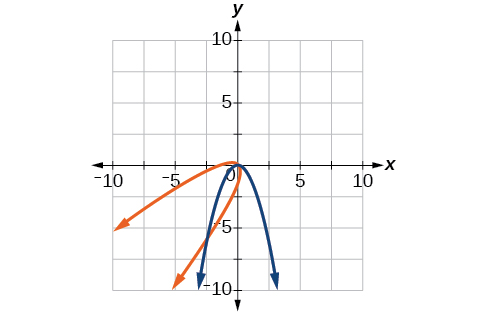$x={y}^{2},\theta ={45}^{\circ }$

$\frac{{x}^{2}}{4}+\frac{{y}^{2}}{1}=1,\theta ={45}^{\circ }$

$\frac{{\left({x}^{\prime }-{y}^{\prime }\right)}^{2}}{8}+\frac{{\left({x}^{\prime }+{y}^{\prime }\right)}^{2}}{2}=1$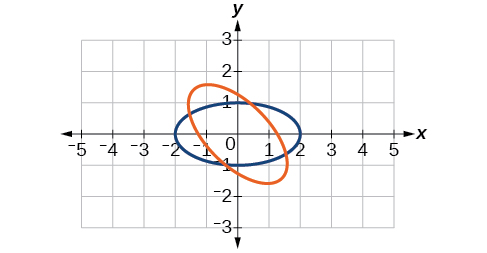$\frac{{y}^{2}}{16}+\frac{{x}^{2}}{9}=1,\theta ={45}^{\circ }$

${y}^{2}-{x}^{2}=1,\theta ={45}^{\circ }$

$\frac{{\left({x}^{\prime }+{y}^{\prime }\right)}^{2}}{2}-\frac{{\left({x}^{\prime }-{y}^{\prime }\right)}^{2}}{2}=1$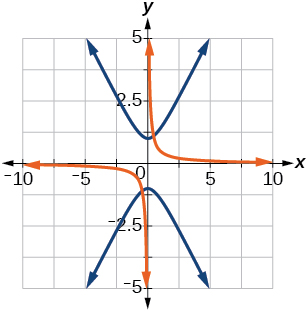$y=\frac{{x}^{2}}{2},\theta ={30}^{\circ }$

$x={\left(y-1\right)}^{2},\theta ={30}^{\circ }$

$\frac{\sqrt{3}}{2}{x}^{\prime }-\frac{1}{2}{y}^{\prime }={\left(\frac{1}{2}{x}^{\prime }+\frac{\sqrt{3}}{2}{y}^{\prime }-1\right)}^{2}$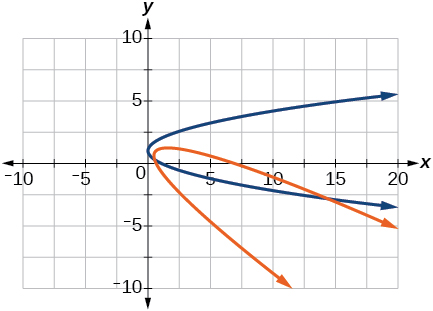$\frac{{x}^{2}}{9}+\frac{{y}^{2}}{4}=1,\theta ={30}^{\circ }$

For the following exercises, graph the equation relative to the $\text{\hspace{0.17em}}{x}^{\prime }{y}^{\prime }\text{\hspace{0.17em}}$ system in which the equation has no $\text{\hspace{0.17em}}{x}^{\prime }{y}^{\prime }\text{\hspace{0.17em}}$ term.

$xy=9$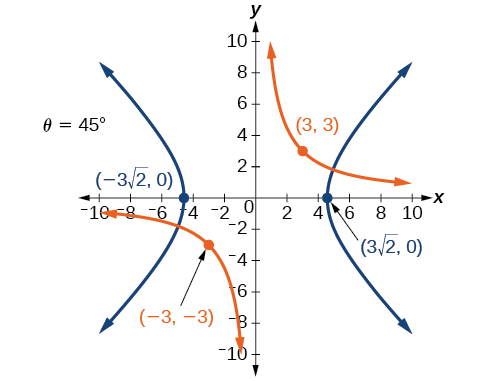${x}^{2}+10xy+{y}^{2}-6=0$

${x}^{2}-10xy+{y}^{2}-24=0$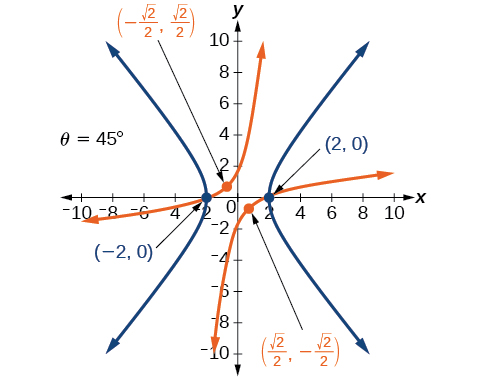$4{x}^{2}-3\sqrt{3}xy+{y}^{2}-22=0$

$6{x}^{2}+2\sqrt{3}xy+4{y}^{2}-21=0$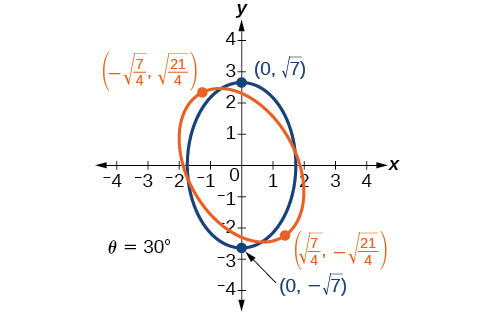$11{x}^{2}+10\sqrt{3}xy+{y}^{2}-64=0$

$21{x}^{2}+2\sqrt{3}xy+19{y}^{2}-18=0$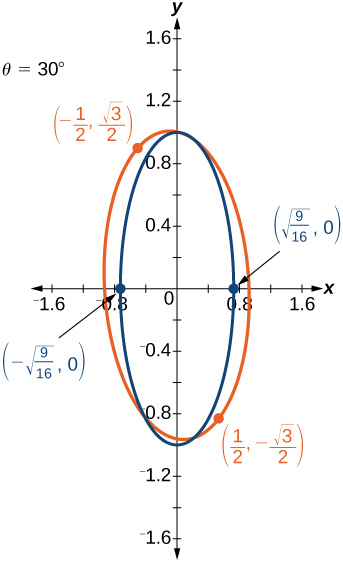$16{x}^{2}+24xy+9{y}^{2}-130x+90y=0$

$16{x}^{2}+24xy+9{y}^{2}-60x+80y=0$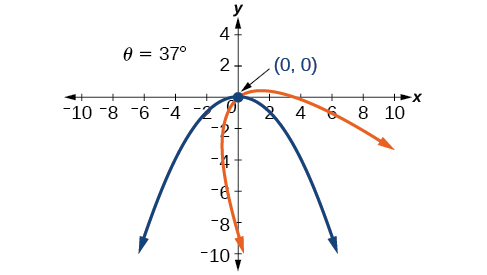$13{x}^{2}-6\sqrt{3}xy+7{y}^{2}-16=0$

$4{x}^{2}-4xy+{y}^{2}-8\sqrt{5}x-16\sqrt{5}y=0$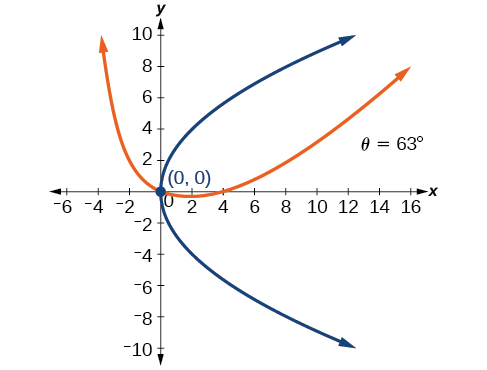For the following exercises, determine the angle of rotation in order to eliminate the $\text{\hspace{0.17em}}xy\text{\hspace{0.17em}}$ term. Then graph the new set of axes.

$6{x}^{2}-5\sqrt{3}xy+{y}^{2}+10x-12y=0$

$6{x}^{2}-5xy+6{y}^{2}+20x-y=0$

$\theta ={45}^{\circ }$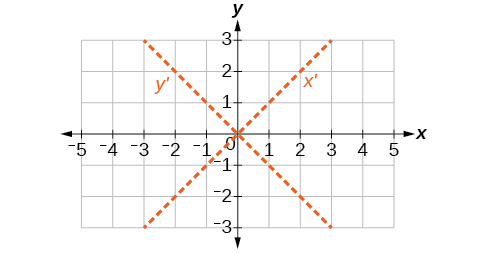$6{x}^{2}-8\sqrt{3}xy+14{y}^{2}+10x-3y=0$

$4{x}^{2}+6\sqrt{3}xy+10{y}^{2}+20x-40y=0$

$\theta ={60}^{\circ }$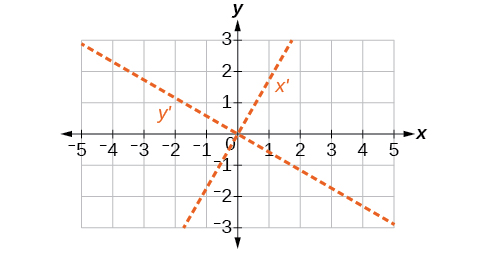$8{x}^{2}+3xy+4{y}^{2}+2x-4=0$

$16{x}^{2}+24xy+9{y}^{2}+20x-44y=0$

$\theta \approx {36.9}^{\circ }$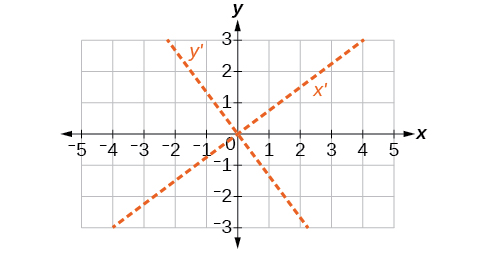For the following exercises, determine the value of $\text{\hspace{0.17em}}k\text{\hspace{0.17em}}$ based on the given equation.

Given $\text{\hspace{0.17em}}4{x}^{2}+kxy+16{y}^{2}+8x+24y-48=0,$ find $\text{\hspace{0.17em}}k\text{\hspace{0.17em}}$ for the graph to be a parabola.

Given $\text{\hspace{0.17em}}2{x}^{2}+kxy+12{y}^{2}+10x-16y+28=0,$ find $\text{\hspace{0.17em}}k\text{\hspace{0.17em}}$ for the graph to be an ellipse.

$-4\sqrt{6}

Given $\text{\hspace{0.17em}}3{x}^{2}+kxy+4{y}^{2}-6x+20y+128=0,$ find $\text{\hspace{0.17em}}k\text{\hspace{0.17em}}$ for the graph to be a hyperbola.

Given $\text{\hspace{0.17em}}k{x}^{2}+8xy+8{y}^{2}-12x+16y+18=0,$ find $\text{\hspace{0.17em}}k\text{\hspace{0.17em}}$ for the graph to be a parabola.

$k=2$

Given $\text{\hspace{0.17em}}6{x}^{2}+12xy+k{y}^{2}+16x+10y+4=0,$ find $\text{\hspace{0.17em}}k\text{\hspace{0.17em}}$ for the graph to be an ellipse.

explain and give four Example hyperbolic function
The denominator of a certain fraction is 9 more than the numerator. If 6 is added to both terms of the fraction, the value of the fraction becomes 2/3. Find the original fraction. 2. The sum of the least and greatest of 3 consecutive integers is 60. What are the valu
1. x + 6 2 -------------- = _ x + 9 + 6 3 x + 6 3 ----------- x -- (cross multiply) x + 15 2 3(x + 6) = 2(x + 15) 3x + 18 = 2x + 30 (-2x from both) x + 18 = 30 (-18 from both) x = 12 Test: 12 + 6 18 2 -------------- = --- = --- 12 + 9 + 6 27 3
Pawel
2. (x) + (x + 2) = 60 2x + 2 = 60 2x = 58 x = 29 29, 30, & 31
Pawel
Mark and Don are planning to sell each of their marble collections at a garage sale. If Don has 1 more than 3 times the number of marbles Mark has, how many does each boy have to sell if the total number of marbles is 113?
Mark = x,. Don = 3x + 1 x + 3x + 1 = 113 4x = 112, x = 28 Mark = 28, Don = 85, 28 + 85 = 113
Pawel
how do I set up the problem?
what is a solution set?
Harshika
find the subring of gaussian integers?
Rofiqul
hello, I am happy to help!
Abdullahi
hi mam
Mark
find the value of 2x=32
divide by 2 on each side of the equal sign to solve for x
corri
X=16
Michael
Want to review on complex number 1.What are complex number 2.How to solve complex number problems.
Beyan
yes i wantt to review
Mark
use the y -intercept and slope to sketch the graph of the equation y=6x
how do we prove the quadratic formular
Darius
hello, if you have a question about Algebra 2. I may be able to help. I am an Algebra 2 Teacher
thank you help me with how to prove the quadratic equation
Seidu
may God blessed u for that. Please I want u to help me in sets.
Opoku
what is math number
4
Trista
x-2y+3z=-3 2x-y+z=7 -x+3y-z=6
can you teacch how to solve that🙏
Mark
Solve for the first variable in one of the equations, then substitute the result into the other equation. Point For: (6111,4111,−411)(6111,4111,-411) Equation Form: x=6111,y=4111,z=−411x=6111,y=4111,z=-411
Brenna
(61/11,41/11,−4/11)
Brenna
x=61/11 y=41/11 z=−4/11 x=61/11 y=41/11 z=-4/11
Brenna
Need help solving this problem (2/7)^-2
x+2y-z=7
Sidiki
what is the coefficient of -4×
-1
Shedrak
the operation * is x * y =x + y/ 1+(x × y) show if the operation is commutative if x × y is not equal to -1ByByBy Caitlyn GobbleBy Sandhills MLTBy Naveen TomarBy Anonymous UserBy Stephanie RedfernBy Cath YuBy OpenStaxBy Anh DaoBy Cameron CaseyBy Brooke Delaney